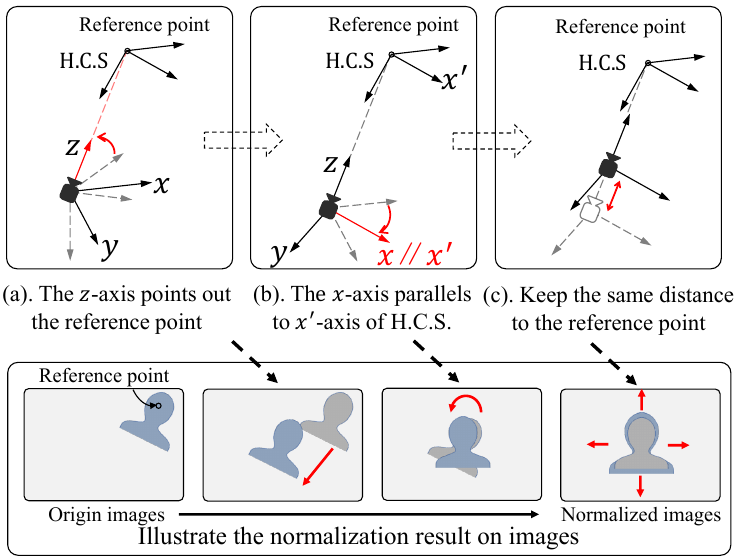# Data rectification

## Introduction

The goal of data rectification is to eliminate the environmental factors by data pre-processing methods and to simplify the gaze regression problem. Current data rectification methods mainly focus on the factors of the head pose and the illumination.

We follow the process of . We assume that the captured eye image is a plane in 3D space, the rotation of the virtual camera can be performed as a perspective transformation on the image. The whole data rectification process is shown in the figure below.
We first define a reference point which is usually eye center or face center. Then, we rotate the virtual camera where the rotated camera is pointing towards the reference point. This operation cancel the variance caused by camera position. We also rotate the virtual camera so that the appearance captured by the rotated cameras is facing the front. Finally, we scale the image to ensure the same distance between subjects and cameras. Note that, the above operation only explain the rectification in image aspect. The gaze direction also should transformed. Please refer our paper for more details.

Illumination also influences the appearance of the human eye. To handle this, researchers usually take gray-scale eye images rather than RGB eye images as input and apply histogram equalization in the gray-scale images to enhance the image.``````@article{Cheng2021Survey,
title={Appearance-based Gaze Estimation With Deep Learning: A Review and Benchmark},
author={Yihua Cheng and Haofei Wang and Yiwei Bao and Feng Lu},
journal={arXiv preprint arXiv:2104.12668},
year={2021}
}
``````

## Tutorial

Please download the code `data_processing_core.py` here.

We also provide an example of normalizing one image.

``````import data_processing_core as dpc
norm = dpc.norm(center = facecenter,
gazetarget = target,
imsize = (224, 224),
camparams = camera)

# Get the normalized face image.
im_face = norm.GetImage(im)

# Crop Right eye images.
llc = norm.GetNewPos(lefteye_leftcorner)
lrc = norm.GetNewPos(lefteye_rightcorner)
im_left = norm.CropEye(llc, lrc)
im_left = dpc.EqualizeHist(im_left)

# Crop Right eye images.
rlc = norm.GetNewPos(righteye_leftcorner)
rrc = norm.GetNewPos(righteye_rightcorner)
im_right = norm.CropEye(rlc, rrc)
im_right = dpc.EqualizeHist(im_right)

# Acquire related info
gaze = norm.GetGaze(scale)
origin = norm.GetCoordinate(facecenter)
rvec, svec = norm.GetParams()
``````

## Document

``````Class norm(center, gazetarget, headrotvec, imsize, camparams, newfocal=960, newdistance=600)
``````

Paramters:

center (narray) : the coordinate of reference point in CCS. The shape is (3, ).

gazetarget (narray) : the coordinate of gaze targets in CCS. The shape is (3, ).

headrotvec (narray) : the rotation vector of head pose in CCS. The shape is (3, ).

imsize (narray) : the image size of normalized images. The shape is (3, ).

camparams (narray) : the camera intrinsics matrix. The shape is (3, ).

newfocal (int or float) : the new focal of camera model.

newdistance (int or float) : the new distance between cameras and subjects.

``````norm.GetParams()
``````

Get the calculted parameters in the normalization. Please refer our paper for the detail algorithm.

Return rvec, svec

rvec (narray) : R in the normaliztion. It is converted into an rotation vector. The shape is (3, ).

svec (narray) : S in the normalization. It only contains the diagonal elements. The shape is (3, )

``````norm.GetImage(image)
``````

Input the origin image and return a normalized image.

Parameters

image (narray) : Original image.

Return im

im (narray) : The normalized image. The size is equal to the `imsize` in `Class norm`.

``````norm.GetCoordinate(coordinate)
``````

Input the coordinate in the origin CCS, output the corresponding coordinate in the normalized CCS.

Parameters

coordinate (narray) : The coordinate in origin CCS. The shape is (3, ).

Return newcoordinate

newcoordinate (narray) : The coordinate in the normalized CCS. The shape is (3, ).

``````norm.GetGaze(scale=True)
``````

Get the gaze direction in the normalized space.

Parameters

scale (bool) : `True` means this code uses the method proposed in . `False` means this code uses the method proposed in .

Return gaze

gaze (narray) : A unit vector of gaze direction.

``````norm.GetHeadRot(vector=True)
``````

Get the head rotation information in the normalized space.

Parameters

vector (bool) : If `True`, return the vector of head orientation. If `False`, return the head rotation matrix.

head (narray) : If `vector=True`, return the vector of head orientation. If `vector=False`, return the head rotation matrix.

``````norm.GetNewPos(pos)
``````

Input the 2D coordinate in the origin image. Output the corresponding 2D coordinate in the normalized image.

Parameters

pos (narray) : The 2D coordinate in the origin image. The shape is (2, ).

Return newpose

newpose (narray): The 2D coordinate in the normalized image. The shape is (2, ).

``````norm.CropEye(lcorner, rcorner)
``````

Input the coordinate of two eye corners. Crop the eye image from the normalized image. Note that, `norm.GetImage(image)` should be called first.

Parameters

lcorner (narray) : The coordinate of left eye corner. The shape is (2,)

lcorner (narray) : The coordinate of right eye corner. The shape is (2,)

Return image

image (narray): the cropped eye image. The shape is (60， 36).

``````norm.CropEyeWithCenter(center)
``````

Input the coordinate of eye center. Crop the eye image from the normalized image. Note that, `norm.GetImage(image)` should be called first.

Parameters

center (narray) : The coordinate of eye corner. The shape is (2,)

Return image

image (narray): the cropped eye image. The shape is (60， 36).

## Reference

 MPIIGaze: Real-World Dataset and Deep Appearance-Based Gaze Estimation

 Learning-by-Synthesis for Appearance-based 3D Gaze Estimation

 Revisiting Data Normalization for Appearance-Based Gaze Estimation